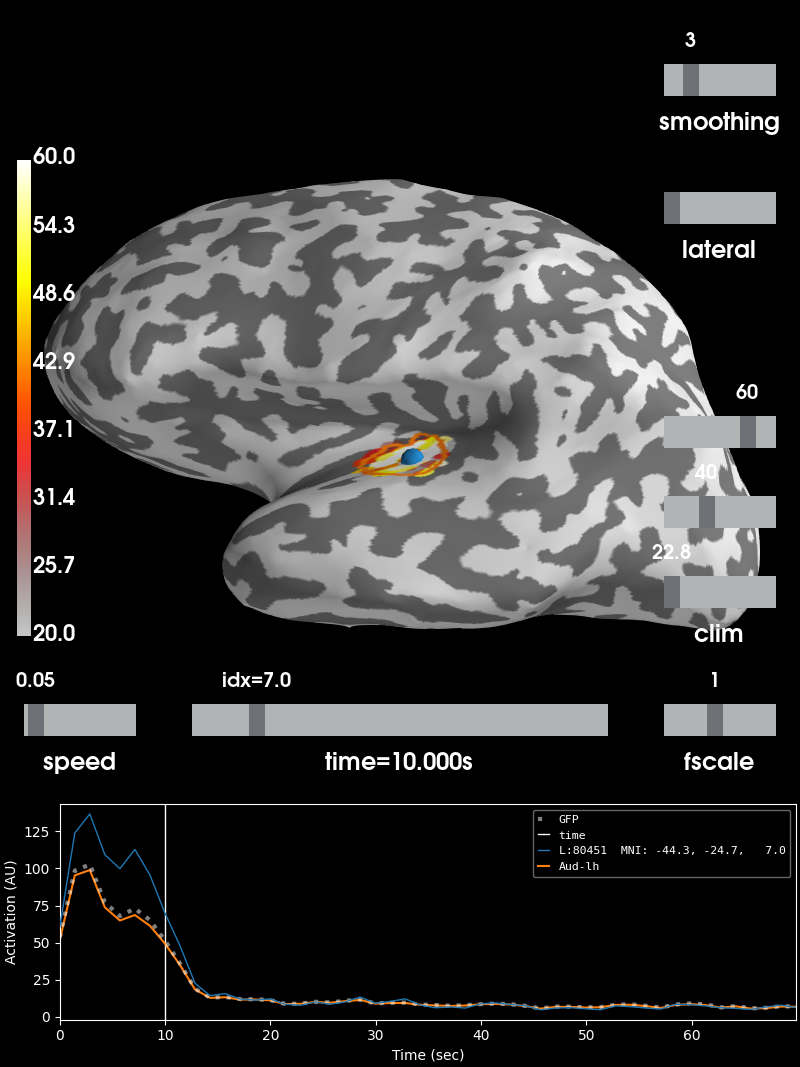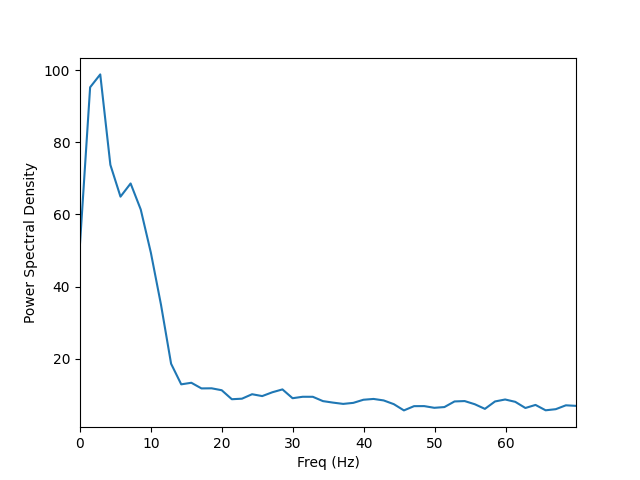# Compute Power Spectral Density of inverse solution from single epochs¶

Compute PSD of dSPM inverse solution on single trial epochs restricted to a brain label. The PSD is computed using a multi-taper method with Discrete Prolate Spheroidal Sequence (DPSS) windows.

```# Author: Martin Luessi <mluessi@nmr.mgh.harvard.edu>
#

import matplotlib.pyplot as plt

import mne
from mne.datasets import sample

print(__doc__)

data_path = sample.data_path()
fname_inv = data_path + '/MEG/sample/sample_audvis-meg-oct-6-meg-inv.fif'
fname_raw = data_path + '/MEG/sample/sample_audvis_raw.fif'
fname_event = data_path + '/MEG/sample/sample_audvis_raw-eve.fif'
label_name = 'Aud-lh'
fname_label = data_path + '/MEG/sample/labels/%s.label' % label_name
subjects_dir = data_path + '/subjects'

event_id, tmin, tmax = 1, -0.2, 0.5
snr = 1.0  # use smaller SNR for raw data
lambda2 = 1.0 / snr ** 2
method = "dSPM"  # use dSPM method (could also be MNE or sLORETA)

# Set up pick list
include = []

# pick MEG channels
picks = mne.pick_types(raw.info, meg=True, eeg=False, stim=False, eog=True,
epochs = mne.Epochs(raw, events, event_id, tmin, tmax, picks=picks,
eog=150e-6))

# define frequencies of interest
fmin, fmax = 0., 70.
bandwidth = 4.  # bandwidth of the windows in Hz
```

Out:

```Reading inverse operator decomposition from /home/circleci/mne_data/MNE-sample-data/MEG/sample/sample_audvis-meg-oct-6-meg-inv.fif...
[done]
[done]
305 x 305 full covariance (kind = 1) found.
Read a total of 4 projection items:
PCA-v1 (1 x 102) active
PCA-v2 (1 x 102) active
PCA-v3 (1 x 102) active
Average EEG reference (1 x 60) active
22494 x 22494 diagonal covariance (kind = 2) found.
22494 x 22494 diagonal covariance (kind = 6) found.
22494 x 22494 diagonal covariance (kind = 5) found.
Did not find the desired covariance matrix (kind = 3)
Computing patch statistics...
[done]
Computing patch statistics...
[done]
Read a total of 4 projection items:
PCA-v1 (1 x 102) active
PCA-v2 (1 x 102) active
PCA-v3 (1 x 102) active
Average EEG reference (1 x 60) active
Source spaces transformed to the inverse solution coordinate frame
Opening raw data file /home/circleci/mne_data/MNE-sample-data/MEG/sample/sample_audvis_raw.fif...
Read a total of 3 projection items:
PCA-v1 (1 x 102)  idle
PCA-v2 (1 x 102)  idle
PCA-v3 (1 x 102)  idle
Range : 25800 ... 192599 =     42.956 ...   320.670 secs
72 matching events found
Setting baseline interval to [-0.19979521315838786, 0.0] sec
Applying baseline correction (mode: mean)
Created an SSP operator (subspace dimension = 3)
3 projection items activated
```

## Compute source space PSD in label¶

..note:: By using “return_generator=True” stcs will be a generator object

instead of a list. This allows us so to iterate without having to keep everything in memory.

```n_epochs_use = 10
stcs = compute_source_psd_epochs(epochs[:n_epochs_use], inverse_operator,
lambda2=lambda2,
method=method, fmin=fmin, fmax=fmax,
bandwidth=bandwidth, label=label,
return_generator=True, verbose=True)

# compute average PSD over the first 10 epochs
psd_avg = 0.
for i, stc in enumerate(stcs):
psd_avg += stc.data
psd_avg /= n_epochs_use
freqs = stc.times  # the frequencies are stored here
stc.data = psd_avg  # overwrite the last epoch's data with the average
```

Out:

```Considering frequencies 0 ... 70 Hz
Preparing the inverse operator for use...
Scaled noise and source covariance from nave = 1 to nave = 1
Created the regularized inverter
Created an SSP operator (subspace dimension = 3)
Created the whitener using a noise covariance matrix with rank 302 (3 small eigenvalues omitted)
Computing noise-normalization factors (dSPM)...
[done]
Picked 305 channels from the data
Computing inverse...
Eigenleads need to be weighted ...
Reducing data rank 99 -> 99
Using 2 tapers with bandwidth 4.0 Hz on at most 10 epochs

0%|          |  : 0/10 [00:00<?,       ?it/s]
10%|#         |  : 1/10 [00:00<00:00,   13.72it/s]
20%|##        |  : 2/10 [00:00<00:00,   12.54it/s]
30%|###       |  : 3/10 [00:00<00:00,   17.30it/s]
50%|#####     |  : 5/10 [00:00<00:00,   19.73it/s]
60%|######    |  : 6/10 [00:00<00:00,   22.40it/s]
80%|########  |  : 8/10 [00:00<00:00,   22.99it/s]
90%|######### |  : 9/10 [00:00<00:00,   24.91it/s]
100%|##########|  : 10/10 [00:00<00:00,   26.74it/s]
100%|##########|  : 10/10 [00:00<00:00,   25.53it/s]
```

Visualize the 10 Hz PSD:

```brain = stc.plot(initial_time=10., hemi='lh', views='lat',  # 10 HZ
clim=dict(kind='value', lims=(20, 40, 60)),
smoothing_steps=3, subjects_dir=subjects_dir)
```Out:

```Extracting time courses for 1 labels (mode: mean)
```

Visualize the entire spectrum:

```fig, ax = plt.subplots()
ax.plot(freqs, psd_avg.mean(axis=0))
ax.set_xlabel('Freq (Hz)')
ax.set_xlim(stc.times[[0, -1]])
ax.set_ylabel('Power Spectral Density')
```Total running time of the script: ( 0 minutes 9.522 seconds)

Estimated memory usage: 15 MB

Gallery generated by Sphinx-Gallery